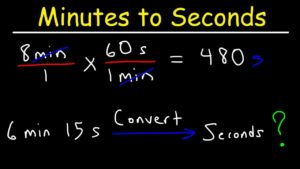# Java: Convert Minutes To Seconds & Vice Versa | 4 Simple Ways

Java program to convert minutes to seconds & seconds to minutes vice versa. The following java code has been written in multiple ways for beginners. If you have any queries just do leave a comment here.

One can safely assume that we all know how many seconds a minute has. Indeed it is a very obvious thing to know. But what if the numbers are too big or if you wanted to try out some other methods apart from having to take the help of calculators.Well, let us delve a bit on the basics as we always love to do and know exactly stuff works.

In the below image one can get a brief idea of how time conversion from minutes to seconds works. In this article, we aim to make these concepts a little bit clearer.

1 minute has 60 seconds in it, let us think of these 60 seconds as a chunk, a compressed value.

So if a chunk of 60 seconds comprises of a minute, 3 of these chunks will make it 3 minutes or 3*60 = 180 seconds.  So if it is asked how many seconds make up for 5 minutes you would say it is 5*60 = 300 seconds.

But what if the total number of minutes was a decimal value? Say 4.5 minutes, again the procedure is the same for that too. Multiply 4.5 with 60 and we get to the total number of seconds. The .5 signifies half a minute or 30 seconds.

Suppose we were to tell how many minutes makeup 3500 seconds. The first thing to do is to divide 3500 by 60. We get the equivalent of 58 minutes or we can say a chunk of 58 chunks of 60 minutes each and the decimal equivalent of 20 seconds, making the value as 58 minutes and 20 seconds.

The same holds up for minutes to hours conversion but that is a story for some another day.

Now that we have learned how exactly do we try it out in standard maths with or without the help of a calculator, let’s move on to do it in a Java program.

## Java Convert Minutes To Seconds – Standard Method

To convert minutes to seconds, we need input minutes which has to be converted to seconds.

This is of integer type which is a primitive datatype so, we make use of Scanner class in Java to read this input at runtime from the console screen. For this, we first create an object instantiating the Scanner class and then call the nextInt() method to read the input minutes (min).

• We know that, 1 min = 60 sec so,
• n mins = n*60 secs by cross multiplication.

So, using the same logic we convert minutes to seconds as shown below and then display it on the console screen.

sec=min*60;

Output:

Using Method

In the earlier method, we can observe that, the entire code is written within the main method only. When we have to make use of the same logic somewhere else in the code at a later stage, we will have to rewrite the code.

Instead, to make the code reusable we place the logic of the code i.e., sec=min*60 in a separate method (seconds) and store this in another variable (sec).

Since, these are outside the main method, we create an object instantiating the class and then my making use of this object, we call the method by passing the input minute (m) as argument.

Also, to display the resultant output stored in the sec variable too we make use of the same object created.

Output:

Using a static method

Above we have noticed that, a separate method was made use of to make a part of code reusable.

But, since it was outside main method object had to be created in the main method.

In place of that, if we make use of static method (minToSec) consisting of the same logic then the need for creating an object is not necessary.

A static method belongs to the class and not the instance, this is the reason for not having the need to create an object.

To this method, we pass our input minutes as parameter and the method returns our desired output seconds which can later be displayed on the console screen.

Output:

### Seconds to Minutes Java Code – Using Command Line

In all the above methods, we have made use of the Scanner class to read primitive datatype input at runtime.

But here, we will be making use of command line arguments to obtain our input.

In this, as we give the run command, we give our input as argument with a space between the run command and the argument.

This argument (args) is taken by the main method but is of String type. Since we require it to be of integer type, we parse it and convert to integer type and store it in a variable (min) as shown below:

This is our necessary input and after getting this, we convert it into seconds by multiply it with 60. The final convert seconds can then be displayed on the console screen using println() method.

x

## Java Program to Add Two Matrices – 4 Ways | Programs

Java program to add two matrices – The following Java Code will let you know ...